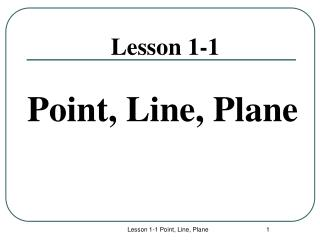DownloadDownload PresentationLesson 1-1

# Lesson 1-1

Télécharger la présentation## Lesson 1-1

- - - - - - - - - - - - - - - - - - - - - - - - - - - E N D - - - - - - - - - - - - - - - - - - - - - - - - - - -
##### Presentation Transcript

1. Lesson 1-1 Point, Line, Plane Lesson 1-1 Point, Line, Plane

2. Definition • A postulate or axiom is an accepted statement of fact. Lesson 1-1 Point, Line, Plane

3. Points • Points do not have actual size. • How to Sketch: Using dots • How to label: Use capital letters Never name two points with the same letter (in the same sketch). A B A C Lesson 1-1 Point, Line, Plane

4. Lines • Lines extend indefinitely and have no thickness or width. • How to sketch : using arrows at both ends. • How to name: 2 ways (1) small script letter – line n (2) any two points on the line - • Never name a line using three points - n A B C Lesson 1-1 Point, Line, Plane

5. Postulate 1-1 • Through any two points there is exactly one line. Lesson 1-1 Point, Line, Plane

6. Intersection of Figures The intersection of two figures is the set of points that are common in both figures. The intersection of two lines is a point. m Line m and line n intersect at point P. P n Continued……. Lesson 1-1 Point, Line, Plane

7. Postulate 1-2 • If two distinct lines intersect, then they intersect at exactly one point. Lesson 1-1 Point, Line, Plane

8. Collinear Points • Collinear points are points that lie on the same line. (The line does not have to be visible.) • A point lies on the line if the coordinates of the point satisfy the equation of the line. Ex: To find if A (1, 0) is collinear with the points on the line y = -3x + 3. Substitute x = 1 and y = 0 in the equation. 0 = -3 (1) + 3 0 = -3 + 3 0 = 0 The point A satisfies the equation, therefore the point is collinear with the points on the line. A B C Collinear C A B Non collinear Lesson 1-1 Point, Line, Plane

9. Planes • A plane is a flat surface that extends indefinitely in all directions. • How to sketch: Use a parallelogram (four sided figure) • How to name: 2 ways (1) Capital script letter – Plane M (2) Any 3 non collinear points in the plane - Plane: ABC/ ACB / BAC / BCA / CAB / CBA A M B C Horizontal Plane Vertical Plane Other Lesson 1-1 Point, Line, Plane

10. Postulate 1-4 • Through any three noncollinear points there is exactly one plane. Lesson 1-1 Point, Line, Plane

11. Different planes in a figure: A B Plane ABCD Plane EFGH Plane BCGF Plane ADHE Plane ABFE Plane CDHG Etc. D C E F H G Lesson 1-1 Point, Line, Plane

12. Other planes in the same figure: Any three non collinear points determine a plane! Plane AFGD Plane ACGE Plane ACH Plane AGF Plane BDG Etc. Lesson 1-1 Point, Line, Plane

13. Coplanar Objects Coplanar objects (points, lines, etc.) are objects that lie on the same plane. The plane does not have to be visible. Are the following points coplanar? A, B, C ? Yes A, B, C, F ? No H, G, F, E ? Yes E, H, C, B ? Yes A, G, F ? Yes C, B, F, H ? No Lesson 1-1 Point, Line, Plane

14. Intersection of Figures The intersection of two figures is the set of points that are common in both figures. The intersection of two lines is a point. m Line m and line n intersect at point P. P n Continued……. Lesson 1-1 Point, Line, Plane

15. 3 Possibilities of Intersection of a Line and a Plane (1) Line passes through plane – intersection is a point. (2) Line lies on the plane - intersection is a line. (3) Line is parallel to the plane - no common points. Lesson 1-1 Point, Line, Plane

16. Intersection of Two Planes is a Line. B P A R Plane P and Plane R intersect at the line Lesson 1-1 Point, Line, Plane

17. Postulate 1-3 • If two distinct planes intersect, then they intersect in exactly one line. Lesson 1-1 Point, Line, Plane